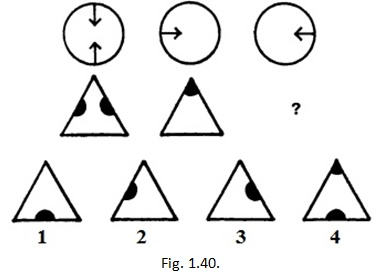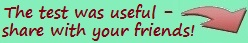https://testy-online.com/eng
Online tests: psychological tests, love and relationships, personality, education and pedagogy, beauty and health, tests for girls, tests in IT disciplines, tests in IT disciplines with answers.Online-testing Studio "AperkotMax"
16 tests in Psychology
1 IT test
Eysenck Test №1 - Determining Your IQ
To pass the test, you have left:
minutes/seconds.

# Eysenck Test №1 - Determining Your IQ. 4 0

There are 40 questions in the test.

The next 40 tasks must be completed in 30 minutes.

### 1. (from 40): Select the correct figure from the four numbered ones (see Fig. 1.1):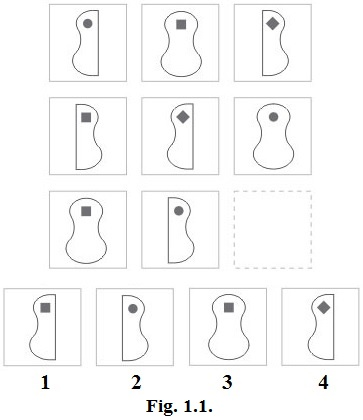а) 1;

b) 2;

c) 3;

d) 4;

### 2. (from 40): Insert the word that completes the first word and begins the second (see Fig. 1.2):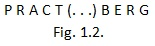### 3. (from 40): Find the odd man out (see Fig. 1.3):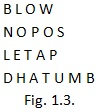### 4. (from 40): Insert the missing number (see Fig. 1.4):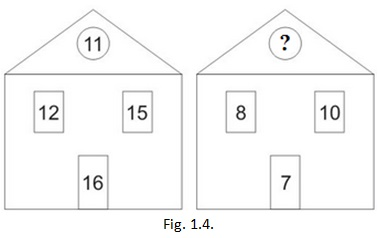### 5. (from 40): Insert the missing word (see Fig. 1.5):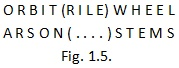### 6. (from 40): Insert the missing number (see Fig. 1.6):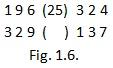### 7. (from 40): What is the next number in the series (see Fig. 1.7)?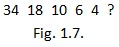### 8. (from 40): Find the odd man out (see Fig. 1.8):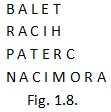### 9. (from 40): Select the correct figure from the numbered ones (see Fig. 1.9):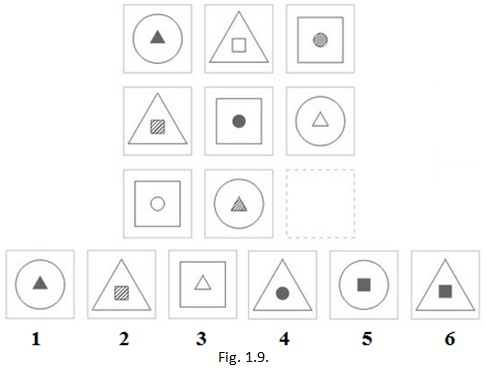а) 1;

b) 2;

c) 3;

d) 4;

e) 5;

f) 6;

### 10. (from 40): Select the correct figure from the six numbered ones (see Fig. 1.10):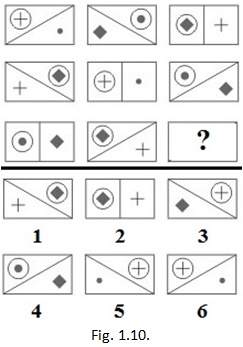а) 1;

b) 2;

c) 3;

d) 4;

e) 5;

f) 6;

### 11. (from 40): Insert the missing letter (see Fig. 1.11):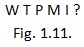### 12. (from 40): Insert the word that completes the first word and begins the second (see Fig. 1.12):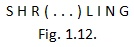### 13. (from 40): Insert the missing number (see Fig. 1.13):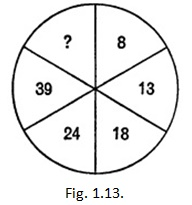### 14. (from 40): Insert the missing number (see Fig. 1.14):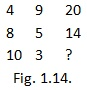### 15. (from 40): Insert the missing number (see Fig. 1.15):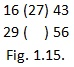### 16. (from 40): Insert the missing letters (see Fig. 1.16):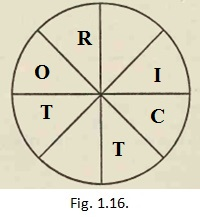### 17. (from 40): Select the correct figure from the numbered ones (see Fig. 1.17):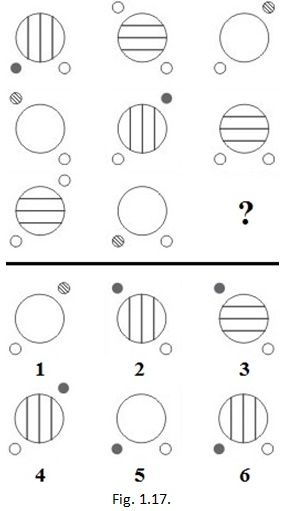а) 1;

b) 2;

c) 3;

d) 4;

e) 5;

f) 6;

### 18. (from 40): Select the correct figure from the six numbered ones (see Fig. 1.18):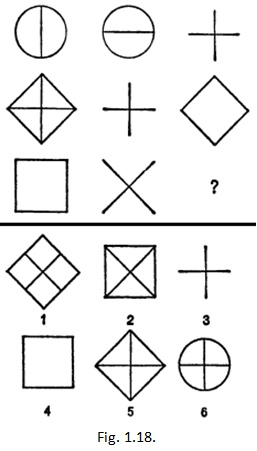а) 1;

b) 2;

c) 3;

d) 4;

e) 5;

f) 6;

### 19. (from 40): Insert the missing number (see Fig. 1.19):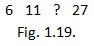### 20. (from 40): Insert the missing number (see Fig. 1.20):### 21. (from 40): Insert the missing word (see Fig. 1.21):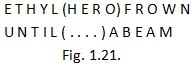### 22. (from 40): Insert the word that completes the first word and begins the second (see Fig. 1.22):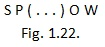### 23. (from 40): Find the odd man out (see Fig. 1.23):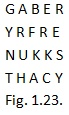### 24. (from 40): Insert the word that means the same as the two words outside the brackets (see Fig. 1.24):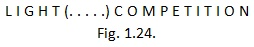### 25. (from 40): Insert the missing letter (see Fig. 1.25):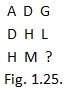### 26. (from 40): Insert the missing letters moving clockwise direction (see Fig. 1.26):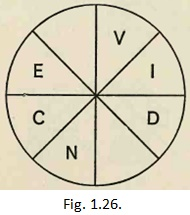### 27. (from 40): Select the correct figure from the six numbered ones (see Fig. 1.27):а) 1;

b) 2;

c) 3;

d) 4;

e) 5;

f) 6;

### 28. (from 40): Select the correct figure from the numbered ones (see Fig. 1.28):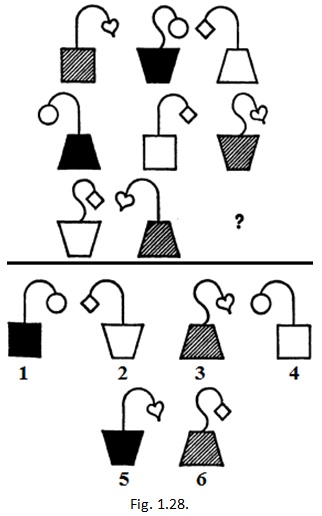а) 1;

b) 2;

c) 3;

d) 4;

e) 5;

f) 6;

### 29. (from 40): Select the correct figure from the six numbered ones (see Fig. 1.29):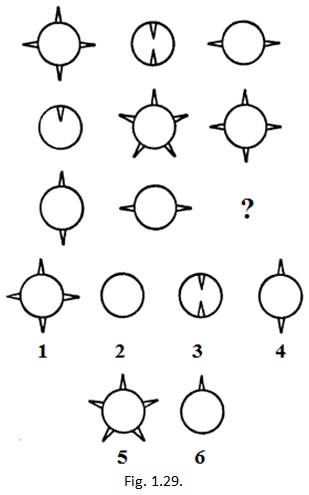а) 1;

b) 2;

c) 3;

d) 4;

e) 5;

f) 6;

### 30. (from 40): Insert the missing word (see Fig. 1.30):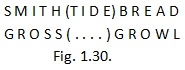### 31. (from 40): Insert the word that means the same as the two words outside the brackets (see Fig. 1.31):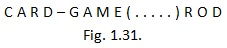### 32. (from 40): Insert the missing number (see Fig. 1.32):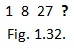### 33. (from 40): Insert the missing word (see Fig. 1.33):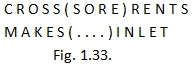### 34. (from 40): Find the odd man out (see Fig. 1.34):### 35. (from 40): Insert the missing letter and number (see Fig. 1.35):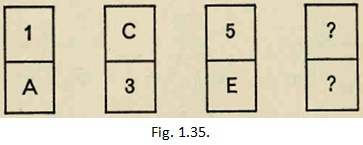### 36. (from 40): Insert the word that means the same as the words outside the brackets (see Fig. 1.36):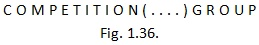### 37. (from 40): Insert the missing word (see Fig. 1.37):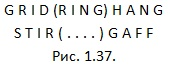### 38. (from 40): Select the correct figure from the six numbered ones (see Fig. 1.38):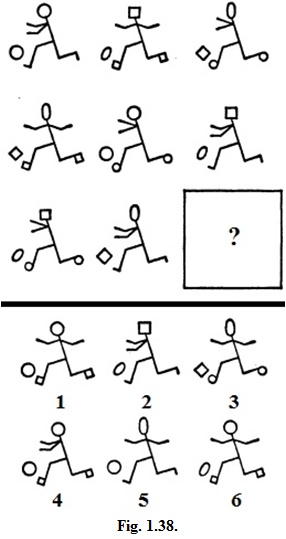а) 1;

b) 2;

c) 3;

d) 4;

e) 5;

f) 6;

### 39. (from 40): Select the correct figure from the six numbered ones (see Fig.1.39):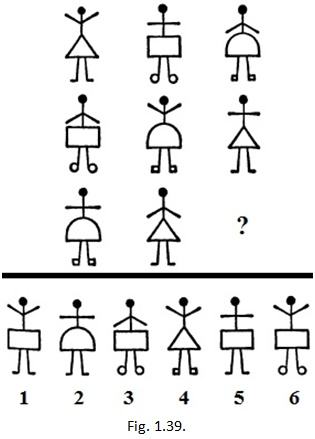а) 1;

b) 2;

c) 3;

d) 4;

e) 5;

f) 6;

### 40. (from 40): Select the correct figure from the four numbered ones (see Fig. 1.40):